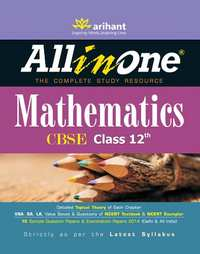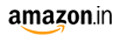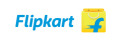Share

# CBSE All in One Mathematics Class 12th (Old Edition) CBSE (Arts) Class 12M.R.P 394/-295.00(25%)400.00-
Product Description

All-in-one Mathematics has been designed for the students studying in Class 12th following the CBSE curriculum. Written by an examiner with years of experience in the subject, this book contains the explanation of various concepts covered under the CBSE Class 12th Mathematics curriculum.

The book provides guidance to the students starting from the stage of learning, to practicing what has been learnt and at last assessing the concepts learnt and practiced. The whole syllabus has been divided into 13 chapters namely, Relations and Functions, Inverse Trigonometric Functions, Matrices, Determinants, Continuity and Differentiability, Application of Derivatives, Integrals, Application of Integrals, Differential Equations, Vector Algebra, Three Dimensional Geometry, Linear Programming and Probability, covering CBSE Class 12th Mathematics syllabus. Book has been designed strictly in sync with the latest syllabus and each chapter has been divided into individual topics for better understanding. Every chapter contains detailed theory supported by illustrations, tables, flow charts, algorithms, notes, remarks, solved examples, worked out problem etc., which will help in effective comprehension of the concepts. The questions given in each chapter have been grouped as Very Short Answer Type, Short Answer Type and Long Answer Type Questions. These questions cover all Previous years' Examination Questions as well as other important questions from the examination point of view. Solutions and explanations to all the questions have been given to facilitate easy learning and understanding. Practice Exercise, a small test that has been given at the end of each chapter which will help the students in assessing their level of understanding. For thorough practice and to give students a real feel of the examination, 10 Sample Question Papers have been given after the chapter wise study. Previous years' CBSE Examination Papers (Delhi and All India) have been given at the end of the book to give the students an insight into the current exam pattern and types of questions asked therein. Also CBSE Mathematics Sample Paper and excerpts from Topper's Answer Sheet have been given at the end of the book. A complete book in itself, this book will serve as a true companion and a guide on your way of achieving highest grades in Class 12 CBSE examination.